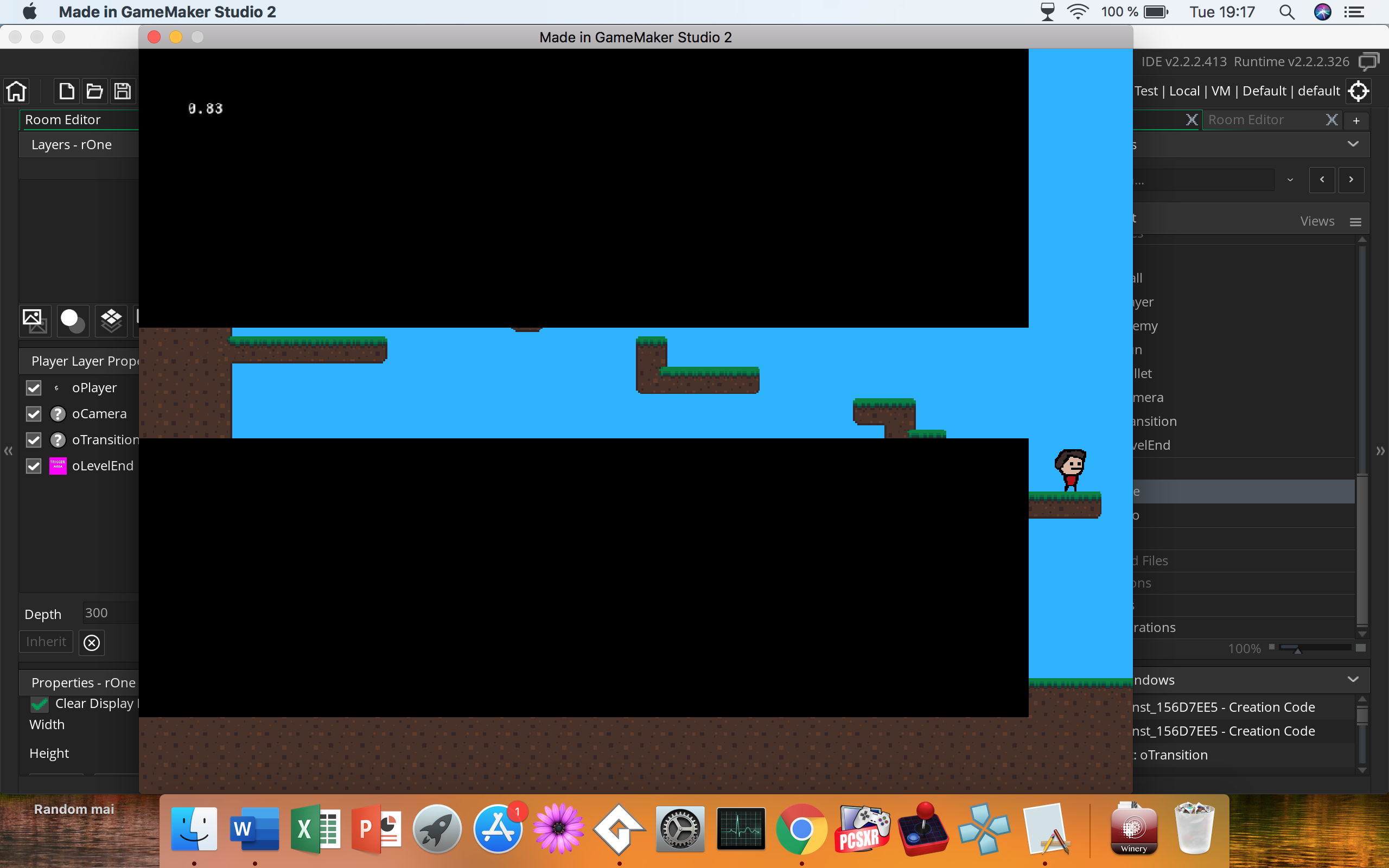##### Member
I am following a Tutorial series of Shaun Spalding.
In the 7th part of his series he explains transitions and I coded it exactly like he did, but for me its not the entire screen.I made an Object for transitions.
in the following Spoilers I paste the code exactly how I wrote it.
///Size variables and mode setup

w = display_get_gui_width();
h = display_get_gui_height();
h_half = h/2;
enum TRANS_MODE
{
OFF,
NEXT,
GOTO,
RESTART,
INTRO
}
mode = TRANS_MODE.INTRO;
percent = 1;
target = room;
///Progress the Transition

if (mode != TRANS_MODE.OFF)
{
if (mode == TRANS_MODE.INTRO)
{
percent = max(0,percent - max((percent/10),0.005));
}
else
{
percent = min(1.2,percent + max(((1.2 - percent)/10),0.005));
}

if (percent == 1.2) || (percent == 0)
{
switch (mode)
{
case TRANS_MODE.INTRO:
{
mode = TRANS_MODE.OFF;
break;
}
case TRANS_MODE.NEXT:
{
mode = TRANS_MODE.INTRO;
room_goto_next();
break;
}
case TRANS_MODE.GOTO:
{
mode = TRANS_MODE.INTRO;
room_goto(target);
break;
}
case TRANS_MODE.RESTART:
{
game_restart();
break;
}
}
}
///Draw Black Bars

if (mode != TRANS_MODE.OFF)
{
draw_set_color(c_black);
draw_rectangle(0,0,w,percent*h_half,false);
draw_rectangle(0,h,w,h-(percent*h_half),false);
}

Here is Shaun Spaldings video I was referring to

I hope someone can help me and thanks in advance#### Sk8dududu

##### Member
I am following a Tutorial series of Shaun Spalding.
In the 7th part of his series he explains transitions and I coded it exactly like he did, but for me its not the entire screen.I made an Object for transitions.
in the following Spoilers I paste the code exactly how I wrote it.
///Size variables and mode setup

w = display_get_gui_width();
h = display_get_gui_height();
h_half = h/2;
enum TRANS_MODE
{
OFF,
NEXT,
GOTO,
RESTART,
INTRO
}
mode = TRANS_MODE.INTRO;
percent = 1;
target = room;
///Progress the Transition

if (mode != TRANS_MODE.OFF)
{
if (mode == TRANS_MODE.INTRO)
{
percent = max(0,percent - max((percent/10),0.005));
}
else
{
percent = min(1.2,percent + max(((1.2 - percent)/10),0.005));
}

if (percent == 1.2) || (percent == 0)
{
switch (mode)
{
case TRANS_MODE.INTRO:
{
mode = TRANS_MODE.OFF;
break;
}
case TRANS_MODE.NEXT:
{
mode = TRANS_MODE.INTRO;
room_goto_next();
break;
}
case TRANS_MODE.GOTO:
{
mode = TRANS_MODE.INTRO;
room_goto(target);
break;
}
case TRANS_MODE.RESTART:
{
game_restart();
break;
}
}
}
///Draw Black Bars

if (mode != TRANS_MODE.OFF)
{
draw_set_color(c_black);
draw_rectangle(0,0,w,percent*h_half,false);
draw_rectangle(0,h,w,h-(percent*h_half),false);
}

Here is Shaun Spaldings video I was referring to

I hope someone can help me and thanks in advanceIf all of your rooms are the same size you can just create a black sprite of that size instead of dealing with all of that code. I can't see the issue with it but it's a lot for something that likely doesn't need it.